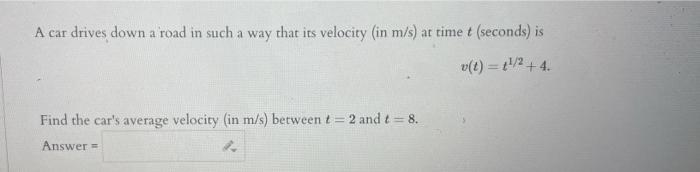# (Solved): A car drives down a road in such a way that its velocity (in $$\mathrm{m} / \mathrm{s}$$ ) at tim ...A car drives down a road in such a way that its velocity (in $$\mathrm{m} / \mathrm{s}$$ ) at time $$t$$ (seconds) is $v(t)=t^{1 / 2}+4$ Find the car's average velocity (in $$\mathrm{m} / \mathrm{s})$$ between $$t=2$$ and $$t=8$$.

We have an Answer from Expert